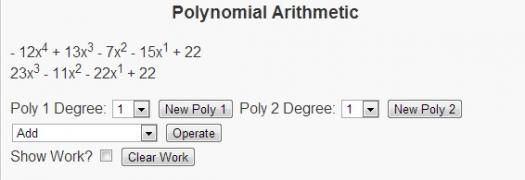# What Do You Know About Arithmetic With Polynomials?

Approved & Edited by ProProfs Editorial Team
The editorial team at ProProfs Quizzes consists of a select group of subject experts, trivia writers, and quiz masters who have authored over 10,000 quizzes taken by more than 100 million users. This team includes our in-house seasoned quiz moderators and subject matter experts. Our editorial experts, spread across the world, are rigorously trained using our comprehensive guidelines to ensure that you receive the highest quality quizzes.
| By Livyn
L
Livyn
Community Contributor
Quizzes Created: 347 | Total Attempts: 144,443
Questions: 10 | Attempts: 150SettingsIn mathematics, a polynomial is an expression consisting of variables (also called indeterminates) and coefficient, that involves only the operations of addition, subtraction, multiplication and non-negative integer exponents of variables.

• 1.

### Polynomials appear in a wide variety of areas of .....

• A.

Mathematics

• B.

Music

• C.

Literature

• D.

Language

A. Mathematics
Explanation
Polynomials appear in a wide variety of areas of mathematics, such as algebra, calculus, and number theory. They are used to represent and solve equations, model real-world phenomena, and analyze mathematical functions. Polynomials are a fundamental concept in mathematics and play a crucial role in various branches of the subject.

Rate this question:

• 2.

### Polynomials also appears in a wide variety of area of ...

• A.

Language

• B.

Science

• C.

Arts

• D.

None

B. Science
Explanation
Polynomials appear in a wide variety of areas in science. They are used to model and solve problems in physics, chemistry, biology, and engineering. In physics, polynomials are used to describe the motion of objects, the behavior of waves, and the forces acting on particles. In chemistry, they are used to calculate reaction rates and equilibrium constants. In biology, polynomials are used to model population growth and enzyme kinetics. In engineering, they are used in electrical circuit analysis, control systems, and signal processing. Therefore, the correct answer is science.

Rate this question:

• 3.

• A.

16th

• B.

12th

• C.

14th

• D.

13th

A. 16th
• 4.

### In ...., Niels Henrik Abel proved the striking result that there are equations of degree 5 whose solutions cannot be expressed by a (finite) formula, involving only arithmetic operations and radicals

• A.

1818

• B.

1999

• C.

1554

• D.

1824

D. 1824
Explanation
In 1824, Niels Henrik Abel proved the result that there are equations of degree 5 whose solutions cannot be expressed by a finite formula involving only arithmetic operations and radicals. This means that there are certain equations of degree 5 that do not have a simple algebraic solution, and their solutions cannot be expressed using a combination of addition, subtraction, multiplication, division, and taking roots. This was a significant discovery in mathematics, highlighting the limitations of algebraic methods in solving certain equations.

Rate this question:

• 5.

### In ...., Évariste Galois proved that most equations of degree higher than four cannot be solved by radicals.

• A.

1830

• B.

1880

• C.

1997

• D.

2000

A. 1830
Explanation
In 1830, Évariste Galois proved that most equations of degree higher than four cannot be solved by radicals. This means that there is no general formula to find the roots of these equations using only the basic operations of addition, subtraction, multiplication, division, and taking roots. Galois' work was groundbreaking and laid the foundation for the development of abstract algebra and Galois theory.

Rate this question:

• 6.

### A real polynomial is a polynomial with real ....

• A.

Slopes

• B.

Coefficient

• C.

Intercepts

• D.

Concavity

B. Coefficient
Explanation
A real polynomial is a polynomial with real coefficients. The coefficients in a polynomial are the numbers multiplied by each variable term. Real coefficients indicate that all the numbers in the polynomial are real numbers, meaning they are not complex or imaginary. Therefore, the correct answer is "coefficient".

Rate this question:

• 7.

### The word polynomial was first used in the ..... century

• A.

17th

• B.

19th

• C.

20th

• D.

18th

A. 17th
Explanation
The word "polynomial" was first used in the 17th century.

Rate this question:

• 8.

### Polynomial is a topic in which course?

• A.

Maths

• B.

Language

• C.

Literature

• D.

Music

A. Maths
Explanation
Polynomial is a topic in mathematics. It involves the study of algebraic expressions consisting of variables and coefficients, combined using addition, subtraction, multiplication, and exponentiation. This topic is commonly taught in math courses as part of algebra or calculus.

Rate this question:

• 9.

### Mathematics is usually studied in ....

• A.

Museum

• B.

Church

• C.

Mosque

• D.

School

D. School
Explanation
Mathematics is usually studied in school because it is a fundamental subject that provides the necessary skills and knowledge for understanding and solving mathematical problems. Schools have dedicated math classes and qualified teachers who can guide students in learning various mathematical concepts and techniques. Additionally, schools provide a structured learning environment with resources such as textbooks, worksheets, and interactive tools that facilitate the learning and practice of mathematics.

Rate this question:

• 10.

### Who is not a mathematician here?

• A.

Will Smith

• B.

Pythagoras

• C.

Albert Einstein

• D.

Isaac Newton

A. Will Smith
Explanation
Will Smith is not a mathematician because he is a well-known actor and rapper, not a mathematician. On the other hand, Pythagoras, Albert Einstein, and Isaac Newton are all renowned mathematicians who have made significant contributions to the field of mathematics.

Rate this question:

Related TopicsBack to top